# evaluating vector space models with word analogies

## Introduction

This post walks through corpus-based methods for evaluating the efficacy of vector space models in capturing semantic relations. Here we consider the standard evaluation tool for VSMs: the offset method for solving word analogies. While this method is not without its limitations/criticisms (see Linzen (2016) for a very nice discussion), our focus here is on an R-based work-flow.

The nuts/bolts of these types of evaluations can often be glossed over in the NLP literature; here we unpack methods and work through some reproducible examples. Ultimately, our goal is to understand how standard VSM parameters (eg, dimensionality & window size) affect model efficacy, specifically for personal and/or non-standard corpora.

## Corpus & model

The corpus used here for demonstration is derived from texts made available via Project Gutenberg. We have sampled the full PG corpus to create a more manageable sub-corpus of ~7K texts and 250M words. A simple description of its construction is available here.

library(tidyverse)
setwd('/home/jtimm/jt_work/GitHub/data_sets/project_gutenberg')
corpus <- readRDS('sample-pg-corpus.rds')

The type of vector space model (VSM) implemented is a GloVe model; the R package text2vec is utilized to construct this semantic space. Below, two text2vec data primitives are created: an iterator and a vectorized vocabulary. See this vignette for a more detailed account of the text2vec framework.

t2v_itokens <- text2vec::itoken(unlist(corpus),
preprocessor = tolower,
tokenizer = text2vec::space_tokenizer,
n_chunks = 1,
ids = names(corpus))

vocab1 <- text2vec::create_vocabulary(t2v_itokens, stopwords = tm::stopwords())
vocab2 <- text2vec::prune_vocabulary(vocab1, term_count_min = 50)
vectorizer <- text2vec::vocab_vectorizer(vocab2)

## Evaluation & analogy

We use two sets of analogy problems for VSM evaluation: the standard Google data set (Mikolov et al. 2013) and the BATS set (Gladkova, Drozd, and Matsuoka 2016). See this brief appendix for some additional details about the problem sets, including category types and examples. See this appendix for a simple code-through of building your own analogy problem sets – compatible structure-wise with the Google data set and the text2vec framework.

I have stored these files on Git Hub, but both are easily accessible online. Important to note, the Google file has not been modified in any way; the BATS file, on the other hand, has been re-structured in the image of the Google file.

questions_file <- paste0(analogy_dir, 'questions-words.txt')
questions_file2 <- paste0(analogy_dir, 'bats-questions-words.txt')

questions_file_path = questions_file,
vocab_terms = vocab2$term)  ## INFO [18:19:44.078] 11779 full questions found out of 19544 total bats_analogy_set <- text2vec::prepare_analogy_questions( questions_file_path = questions_file2, vocab_terms = vocab2$term) 
## INFO  [18:19:45.217] 39378 full questions found out of 56036 total
tests <- c(google_analogy_set, bats_analogy_set)

Long & short of the vector offset method applied to analogy problems. Per some analogy defined as (1) below:

(1)  a:a* :: b:__

where a = Plato, a* = Greek, and b = Copernicus, we solve for b* as

(2) b* = a* - a + b 

based on the assumption that:

(3) a* - a = b* - b

In other words, we assume that the vector offsets between two sets of words related semantically in similar ways will be consistent when plotted in 2d semantic space. Solving for b*, then, amounts to identifying the word whose vector representation is most similar (per cosine similarity) to a* - a + b (excluding a*, a, or b).

## Experimental set-up

### Parameters

So, to evaluate effects of window size and dimensionality on the efficacy of a GloVe model in solving analogies, we build a total of 50 GloVe models – ie, all combinations of window sizes 3:12 and model dimensions in (50, 100, 150, 200, 250).

p_windows <- c(3:12)
p_dimensions <- c(50, 100, 150, 200, 250)

ls <- length(p_windows) * length(p_dimensions)
z = 0
results <- list()
details <- vector()

### Flow

The nasty for loop below can be translated into layman’s terms as: for window size j and dimensions k, (1) build GloVe model j-k via text2vec::GlobalVectors, and then (2) test accuracy of GloVe model j-k via text2vec::check_analogy_accuracy.

for(j in 1:length(p_windows)) {

tcm <- text2vec::create_tcm(it = t2v_itokens,
vectorizer = vectorizer,
skip_grams_window = p_windows[j])

for(k in 1:length(p_dimensions)) {

glove <- text2vec::GlobalVectors$new(rank = p_dimensions[k], x_max = 10) wv_main <- glove$fit_transform(tcm,
n_iter = 10,
convergence_tol = 0.01)
glove_vectors <- wv_main + t(glove$components) res <- text2vec::check_analogy_accuracy( questions_list = google_analogy_set, m_word_vectors = glove_vectors) id <- paste0('-windows_', p_windows[j], '-dims_', p_dimensions[k]) z <- z + 1 results[[z]] <- res details[z] <- id } } ### Output structure Responses to the analogy test are summarized as a list of data frames – one for each of our 50 GloVe models. names(results) <- details answers <- results %>% bind_rows(.id = 'model') Test components have been hashed (per text2vec) to speed up the “grading” process – here, we cross things back to actual text. key <- vocab2 %>% mutate(id = row_number()) %>% select(id, term) tests_df <- lapply(tests, data.frame) %>% bind_rows() %>% mutate(aid = row_number()) tests_df$X1 <- key$term[match(tests_df$X1, key$id)] tests_df$X2 <- key$term[match(tests_df$X2, key$id)] tests_df$X3 <- key$term[match(tests_df$X3, key$id)] tests_df$X4 <- key$term[match(tests_df$X4, key$id)] Then we join test & response data to create a single, readable data table. predicted_actual <- answers %>% group_by(window, dimensions) %>% mutate(aid = row_number()) %>% ungroup() %>% left_join(key, by = c('predicted' = 'id')) %>% rename(predicted_term = term) %>% left_join(key, by = c('actual' = 'id')) %>% rename(actual_term = term) %>% left_join(tests_df %>% select(aid, X1:X3)) %>% na.omit %>% mutate(correct = ifelse(predicted == actual, 'Y', 'n')) A sample of this table is presented below. incorrect answers are generally more interesting. ## Results: model parameters Our performance metric for a given model, then, is the percentage of correct analogy responses, or analogy accuracy. Accuracy scores by dimensions, window size, and data set/analogy category are computed below. mod_category_summary <- answers %>% mutate(correct = ifelse(predicted == actual, 'Y', 'N')) %>% mutate(dset = ifelse(grepl('gram', category), 'google', 'bats')) %>% group_by(dset, dimensions, window, category, correct) %>% summarize(n = n()) %>% ungroup() %>% spread(correct, n) %>% mutate(N = as.integer(N), Y = as.integer(Y),) ### Vector dimensionality effect mod_summary <- mod_category_summary %>% filter(!is.na(Y)) %>% # group_by(dset, window, dimensions) %>% summarize(N = sum(N), Y = sum(Y)) %>% ungroup() %>% mutate (per = round(Y/(N+Y) *100, 1))  The plot below illustrates the relationship between analogy accuracy and # of model dimensions as a function of window size, faceted by analogy set. Per plot, GloVe model gains in analogy performance plateau at 150 dimensions for all window sizes; in several instances, accuracy decreases at dimensions > 150. Also – the BATS collection of analogies would appear to be a bit more challenging. mod_summary %>% ggplot() + geom_line(aes(x = dimensions, y = per, color = factor(window), linetype = factor(window)), size = 1) + facet_wrap(~dset) + theme_minimal() + ggthemes::scale_color_stata() + theme(axis.text.x = element_text(angle = 90, hjust = 1)) + theme(legend.position = 'right') + ylab('Accuracy (%)') + xlab("Dimensions") + labs(title = 'Accuracy (%) versus # Dimensions')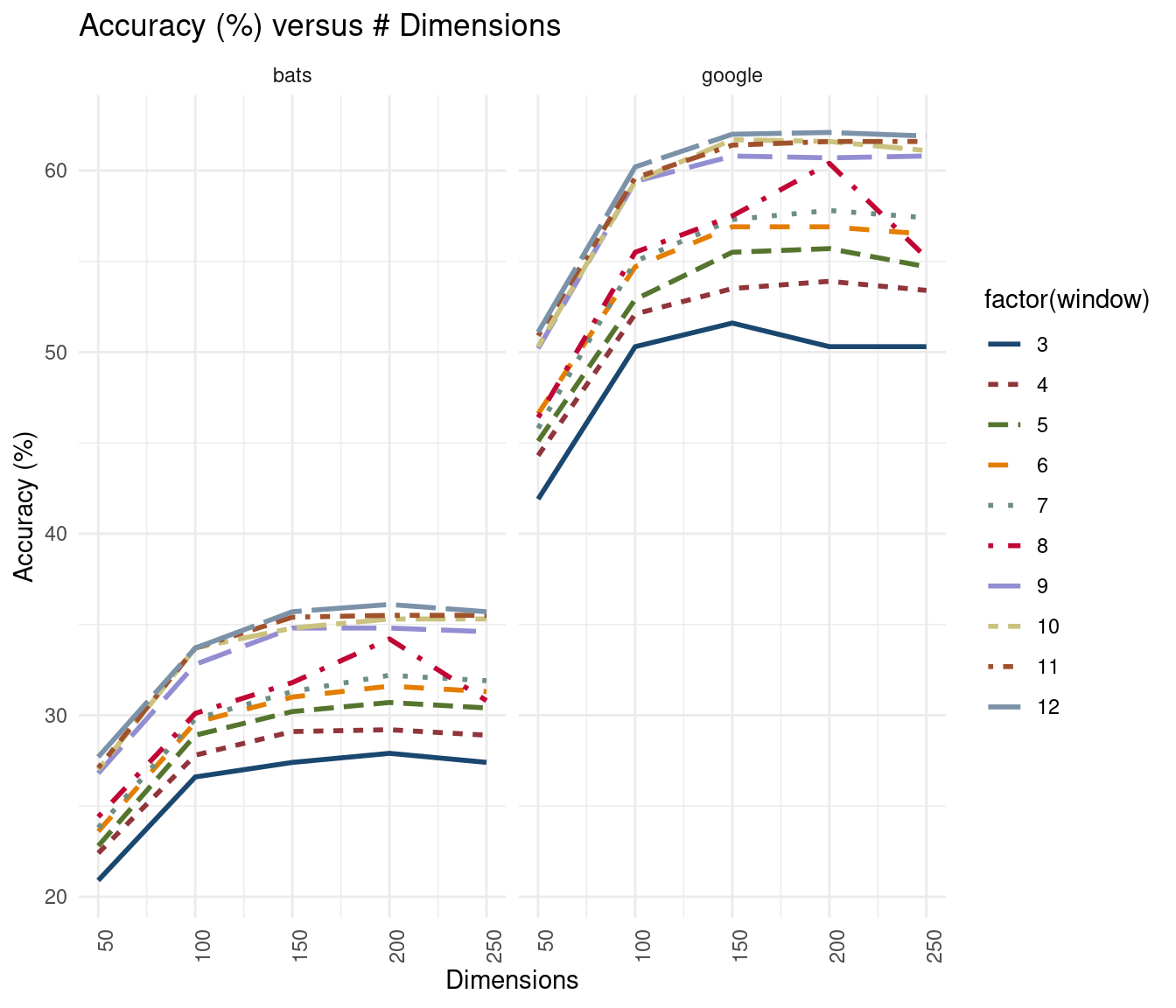### Window Size effect The plot below illustrates the relationship between analogy accuracy and window size as a function of dimensionality. Here, model performance improves per step-increase in widow size – accuracy seems to improve most substantially from window sizes 8 to 9. Also, some evidence of a leveling off at window sizes > 9 for higher-dimension models. The simplest and highest performing model, then, for this particular corpus (in the aggregate) is a window size = 10 and dimensions = 150 model. mod_summary %>% ggplot() + geom_line(aes(x = window, y= per, color = factor(dimensions), linetype = factor(dimensions) ), size = 1.25) + facet_wrap(~dset) + theme_minimal() + ggthemes::scale_color_few() + scale_x_continuous(breaks=c(3:12)) + theme(legend.position = 'right') + ylab('Accuracy (%)') + xlab("Window Size") + labs(title = 'Accuracy (%) versus Window Size')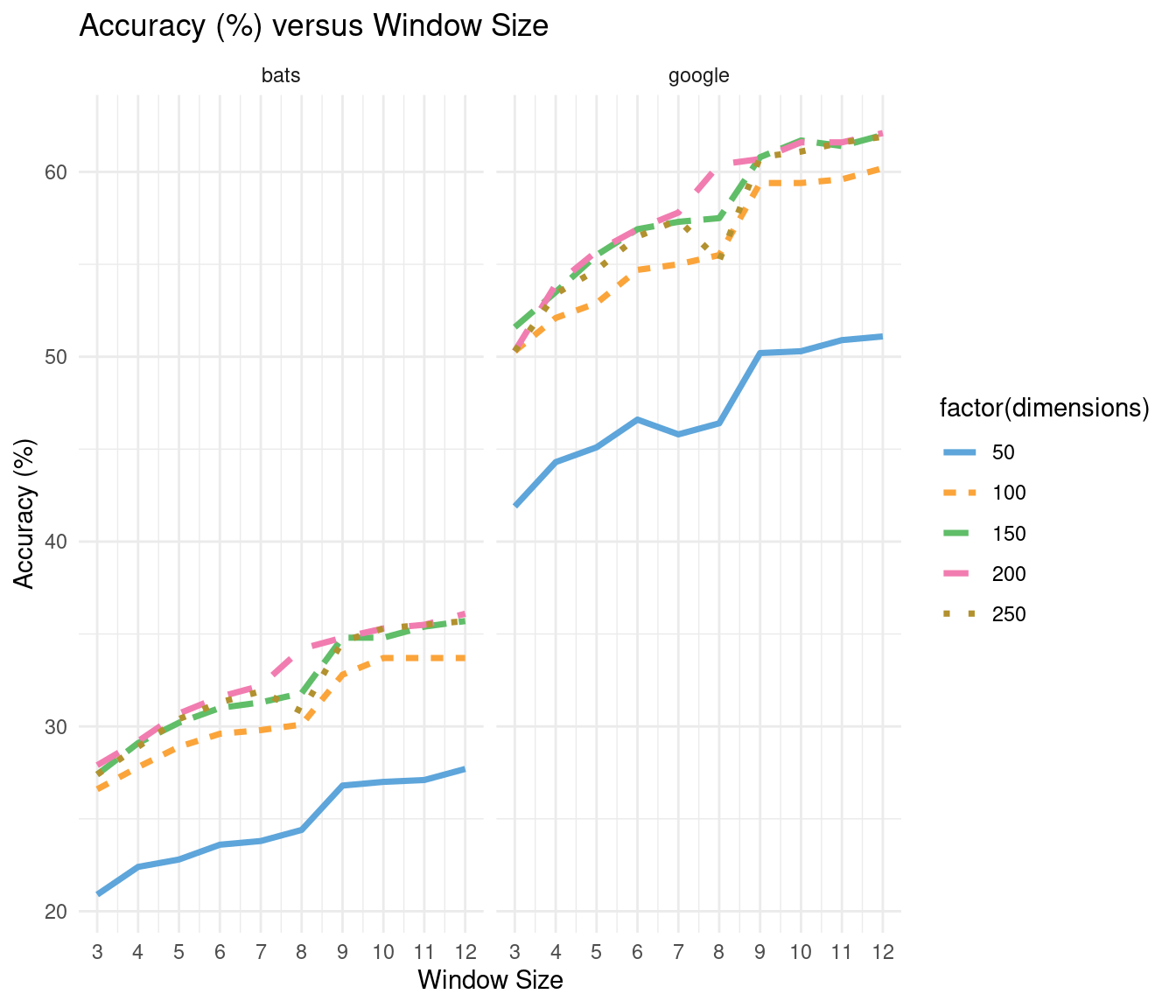## Results: analogy categories Next, we disaggregate model efficacy by analogy category and window size, holding dimensionality constant at 150. Results for each set of analogy problems are visualized as tiled “heatmaps” below; dark green indicates higher accuracy within a particular category, dark brown lower accuracy. Folks have noted previously in the literature that smaller window sizes tend to be better at capturing relations more semantic (as opposed to more grammatical) in nature. Some evidence for that here. ### Google analogy set mod_category_summary %>% filter(!grepl('_', category)) %>% filter(dimensions == 150) %>% mutate(per = round(Y/(N+Y) *100, 1)) %>% filter(!is.na(per)) %>% group_by(category) %>% mutate(rank1 = rank(per)) %>% ungroup() %>% ggplot(aes(x = factor(window), y = category)) + geom_tile(aes(fill = rank1)) + geom_text(aes(fill = rank1, label = per), size = 3) + scale_fill_gradient2(low = scales::muted("#d8b365"), mid = "#f5f5f5", high = scales::muted('#5ab4ac'), midpoint = 5) + theme(legend.position = 'none') + xlab('WINDOW SIZE') + ggtitle('Google analogies: accuracy by category')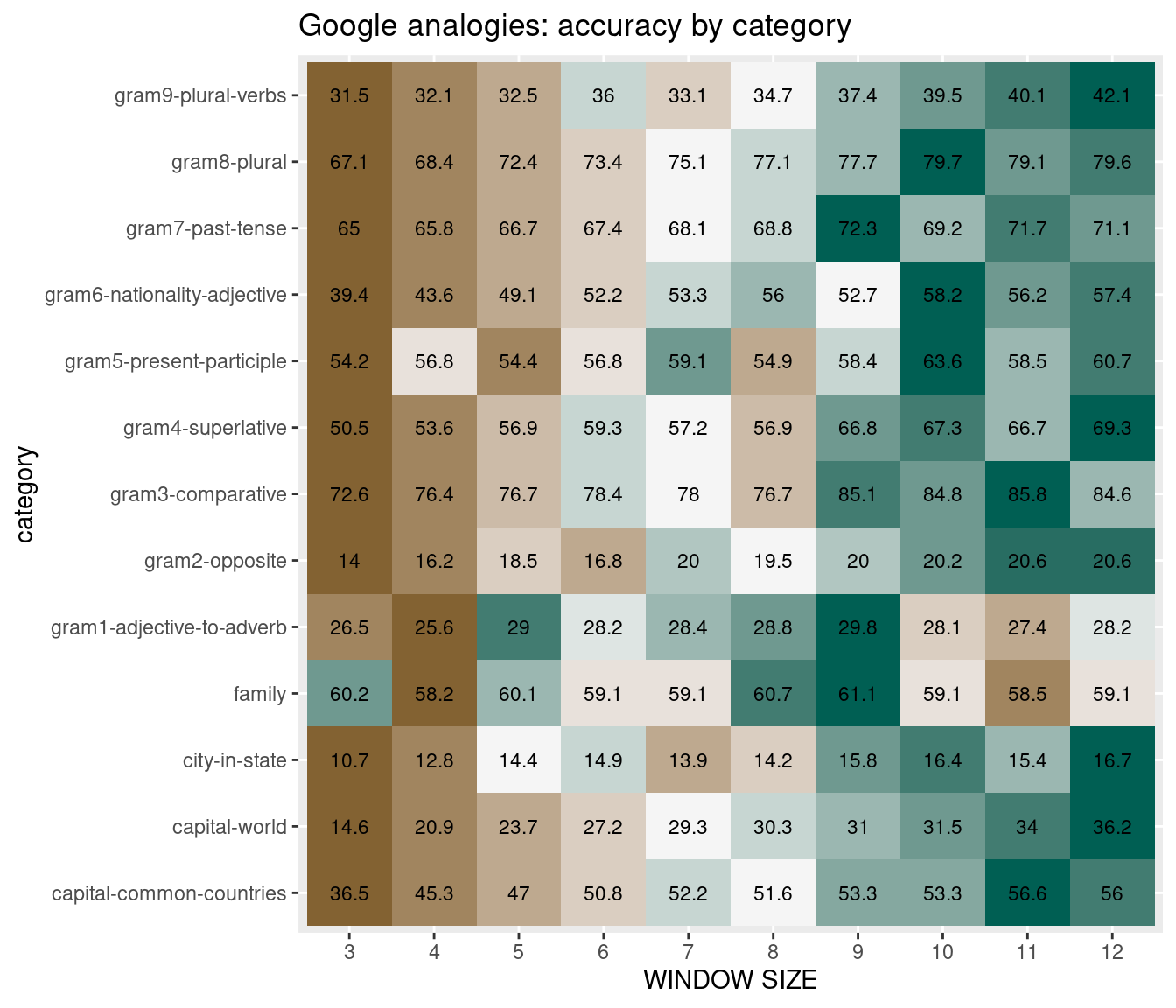### BATS analogy set mod_category_summary %>% filter(grepl('_', category)) %>% filter(dimensions == 150) %>% mutate(per = round(Y/(N+Y) *100, 1), category = gsub('^.*/','', category)) %>% filter(!is.na(per)) %>% group_by(category) %>% mutate(rank1 = rank(per)) %>% ungroup() %>% ggplot(aes(x = factor(window), y = category)) + geom_tile(aes(fill = rank1)) + geom_text(aes(fill = rank1, label = per), size = 3) + scale_fill_gradient2(low = scales::muted("#d8b365"), mid = "#f5f5f5", high = scales::muted('#5ab4ac'), midpoint = 5) + theme(legend.position = 'none') + xlab('WINDOW SIZE') + ggtitle('BATS analogies: accuracy by category')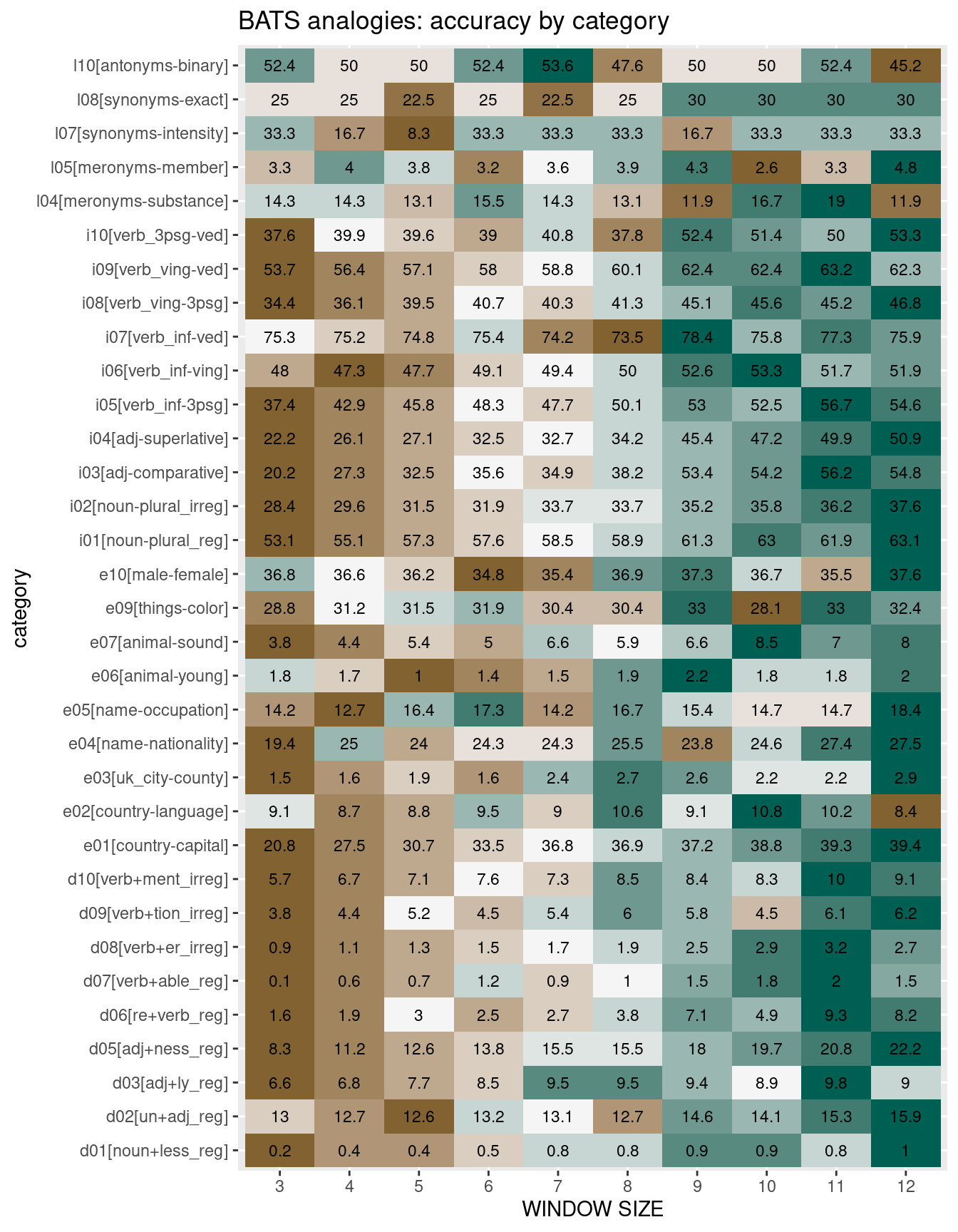## Visualizing vector offsets ### GloVe model in two dimensions For demonstration purposes, we use a semantic space derived from the window size = 5 and dimensions = 100 GloVe model. This space is transformed from 100 GloVe dimensions to two dimensions via principal component analysis. pca_2d <- prcomp(glove_vectors, scale = TRUE, center = TRUE) %>% pluck(5) %>% data.frame() %>% select(PC1, PC2) A 30,000 foot view of this two-dimensional semantic space. ggplot(data = pca_2d, aes(x = PC1, y = PC2)) + geom_point(size = .05, color = 'lightgray') + geom_text(data = pca_2d, aes(x = PC1, y = PC2, label = rownames(pca_2d)), size = 3, color = 'steelblue', check_overlap = TRUE) + xlim (-2.5,2.5) + ylim(-2.5,2.5) + theme_minimal()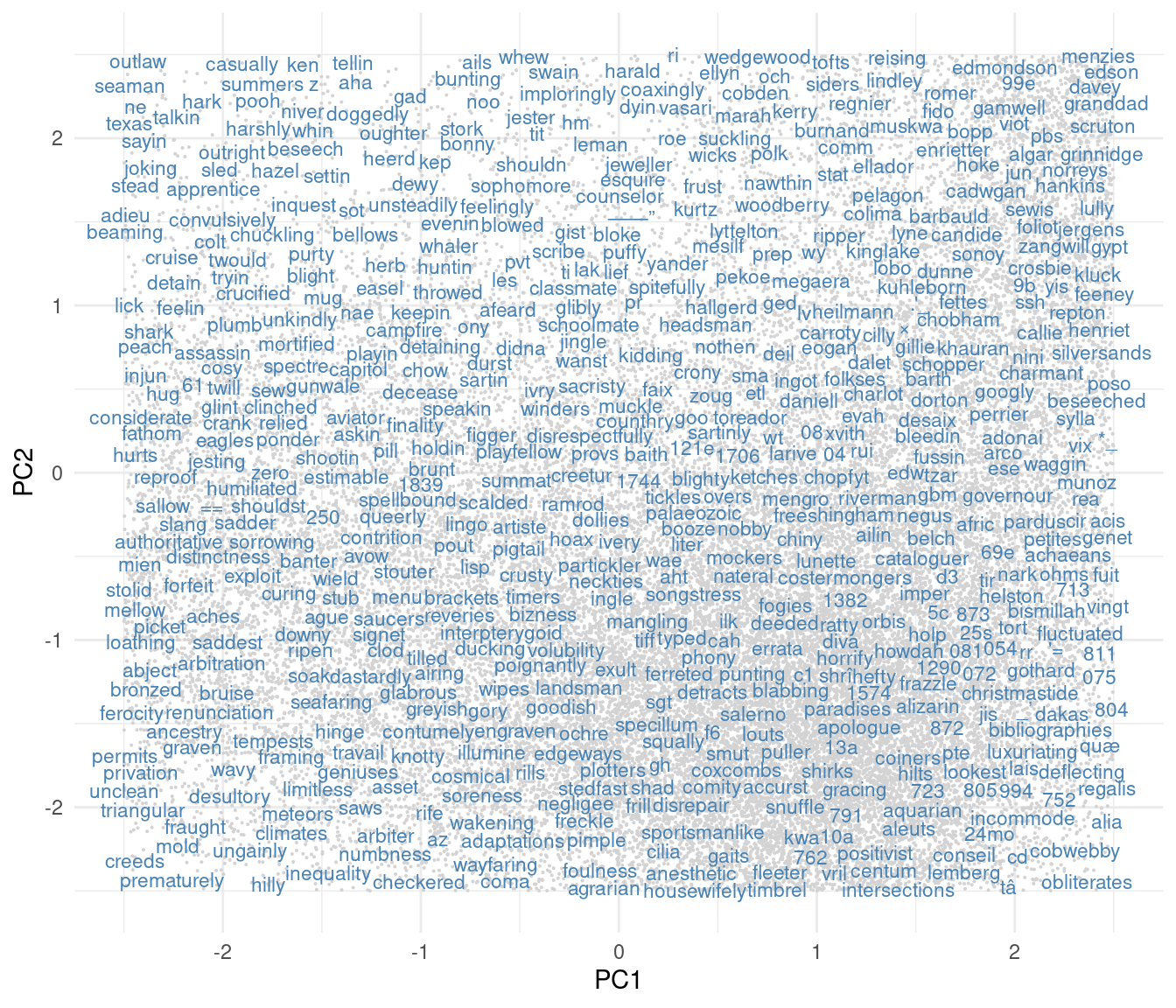### Copernicus & Plato x1 = 'copernicus'; x2 = 'polish'; y1 = 'plato'; y2 = 'greek' y <- pca_2d[rownames(pca_2d) %in% c(x1, x2, y1, y2),] Finally, a visual demonstration of the vector offset method at-work in solving the Copernicus analogy problem. Situated within the full semantic space for context. ggplot(data = pca_2d, aes(x = PC1, y = PC2)) + geom_text(data = pca_2d, aes(x = PC1, y = PC2, label = rownames(pca_2d)), size = 3, color = 'gray', check_overlap = TRUE) + xlim(min(c(off_dims$x1, off_dims$x2)), max(c(off_dims$x1, off_dims$x2))) + ylim(min(c(off_dims$y1, off_dims$y2)), max(c(off_dims$y1, off_dims\$y2))) +
geom_segment(data = off_dims[1:2,],
aes(x = x2, y = y2,
xend = x1, yend = y1),
color = '#df6358',
size = 1.25,
arrow = arrow(length = unit(0.025, "npc"))) +
geom_segment(data = off_dims[3:4,],
aes(x = x1, y = y1,
xend = x2, yend = y2),
color = 'steelblue',
size = 1.25,
#linetype = 4,
arrow = arrow(length = unit(0.025, "npc"))) +
ggrepel::geom_text_repel(
data  = y,
aes(label = toupper(rownames(y))),
direction = "y",
hjust = 0,
size = 4.25,
color = 'black') +
theme_minimal() +
ggtitle(paste0(x1, ':', x2, ' :: ', y1, ':', y2))## Summary & caveats

Mostly an excuse to gather some thoughts. I use GloVe models quite a bit for exploratory purposes. To better trust insights gained from exploration, it is generally nice to have an evaluative tool, however imperfect. And certainly to justify parameter selection for corpus-specific tasks. Hopefully a useful resource and guide. For some innovative and more thoughtful applications of VSMs, see Weston et al. (2019) and Tshitoyan et al. (2019).